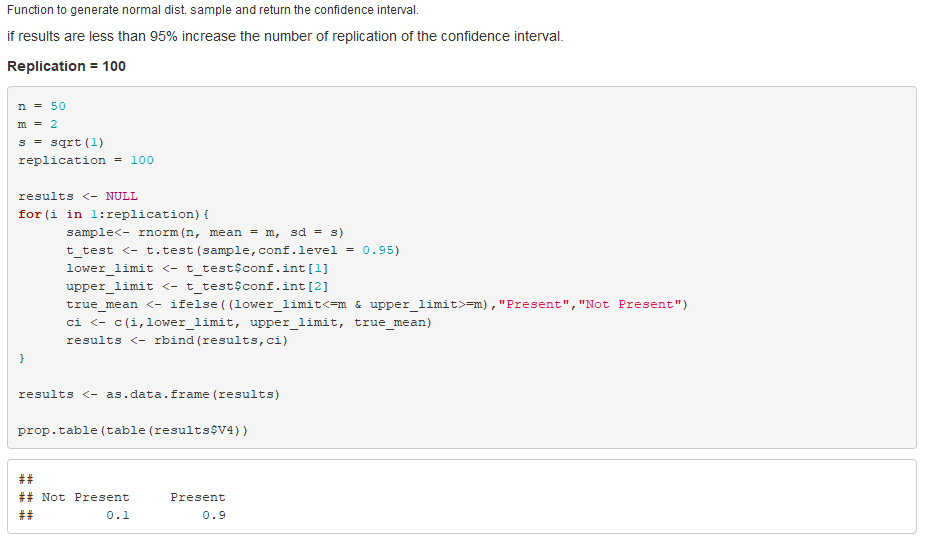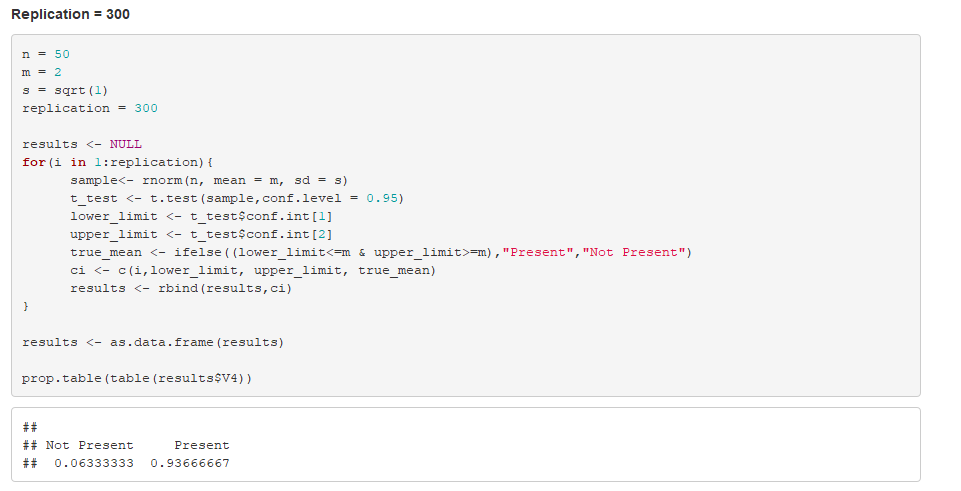##### Question

In: Statistics and Probability

# Using built in R functions to calculate mean and standard deviation. Simulate the X vector with...

Using built in R functions to calculate mean and standard deviation. Simulate the X vector with a length of 50 from a normal distribution with mean 2 and variance 1. Using a for() loop, create 100 different confidence intervals and also create a variable that keeps a count of how many of these confidence intervals contain the true mean 2. Hint: Use If/else statement to keep track of whether the confidence interval contains the true mean or not within the loop. Use set.seed(i) (i.e., i in 1:100) to make sure that other people can reproduce your results and also you have different results for different replications. Print (count/100) at the end. If your solution is less than 95%, what actions can you do to improve the results? Demonstrate it using R.

## Solutions

##### Expert Solution

R code given below## Related Solutions

##### Using the table below, calculate the variance of X, variance of Y, standard deviation of X,...
Using the table below, calculate the variance of X, variance of Y, standard deviation of X, standard deviation of Y,   the covariance between X and Y, the correlation coefficient between X and Y, and find the regression line assuming X is the independent variable (find the slope and the intercept). Show your work Fill in the Blanks: X Y X-Xbar Y-Ybar (X-Xbar)^2 (Y-Ybar)^2 (X-Xbar)*(Y-Ybar) 5 3 9 6 12 15 17 20 21 23 Sum Calculate the following and show...
##### This data is from a sample. Calculate the mean, standard deviation, and variance. x 38.3 35.2...
This data is from a sample. Calculate the mean, standard deviation, and variance. x 38.3 35.2 26.7 49.9 44.6 14.2 16.4 34 14.4 Please show the following answers to 2 decimal places. Sample Mean = Sample Standard Deviation = Sample Variance = Ooops - now you discover that the data was actually from a population! So now you must give the population standard deviation. Population Standard Deviation =
##### Using Minitab, calculate the mean, standard deviation, variance, and median of the following four data sets....
Using Minitab, calculate the mean, standard deviation, variance, and median of the following four data sets. Comment on the results. A B C D 100 50 50 75 100 75 100 75 100 100 100 75 100 125 100 100 100 150 150 175
##### Use R functions search and objects to find all possible R built-in functions related to exp...
Use R functions search and objects to find all possible R built-in functions related to exp distribution. rexp should be one of them. dexp is another one. Explain what the following two lines of codes do: curve(dexp, from=0, to=4)
##### Movies 3a and 3b: 1. Using either R studio and/or SPSS calculate the mean and standard...
Movies 3a and 3b: 1. Using either R studio and/or SPSS calculate the mean and standard deviation for the question 4 data (first tab in your excel spreadsheet). Be sure to cut and paste your output from SPSS and/or your R code into this document. (1 point) The standard deviation was: The mean was: Age 5 3 5 3 2 3 1 2 4 2 4 2 6 5 5 4 3 5 3 1 4 2 3 5 6...
##### Calculate the flux of the vector field F? (r? )=9r? , where r? =?x,y,z?, through a...
Calculate the flux of the vector field F? (r? )=9r? , where r? =?x,y,z?, through a sphere of radius 4 centered at the origin, oriented outward. Flux =
##### Calculate the standard deviation of the following test data by hand. Describe the mean and standard...
Calculate the standard deviation of the following test data by hand. Describe the mean and standard deviation in words after calculating it. Record the steps. Test Scores: 40, 25,50, 30, 60, 80, 20, 70
##### 3. R has a built-in character vector of US State names, state.name. Use this character vector...
3. R has a built-in character vector of US State names, state.name. Use this character vector and R's character functions to answer the following questions.Show R code (a) List all the US State names that are more than one word. How many are there? (b) What is the longest US State name(s) (including spaces) and how long is it? (c) What is the longest single word US State name and how long is it? (d) List all the US State...
##### Calculate (by hand) the mean and standard deviation for the binomial distribution with the probability of...
Calculate (by hand) the mean and standard deviation for the binomial distribution with the probability of a success being 0.50 and n = 10. Either show work or explain how your answer was calculated. Use these formulas to do the hand calculations: Mean = np, Standard Deviation =  Comparison: Calculate (by hand) the mean and standard deviation for the binomial distribution with the probability of a success being 0.10 and n = 10. Write a comparison of these statistics to those...
##### Let C(R) be the vector space of continuous functions from R to R with the usual...
Let C(R) be the vector space of continuous functions from R to R with the usual addition and scalar multiplication. Determine if W is a subspace of C(R). Show algebraically and explain your answers thoroughly. a. W = C^n(R) = { f ∈ C(R) | f has a continuous nth derivative} b. W = {f ∈ C^2(R) | f''(x) + f(x) = 0} c. W = {f ∈ C(R) | f(-x) = f(x)}.